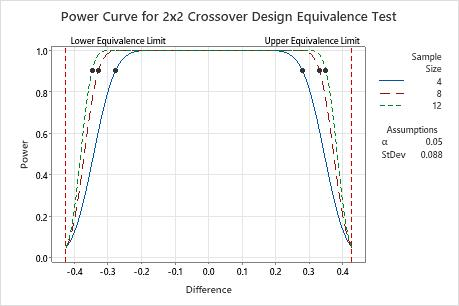# Interpret the key results for Power and Sample Size for 2x2 Crossover Design Equivalence Test

Complete the following steps to interpret power and sample size for a 2x2 crossover design equivalence test. Key output includes the difference, the sample size, the power, and the power curve.

## Step 1: Examine the calculated values

Using the values of the two properties that you entered, Minitab calculates the difference, the sample size, or the power of the test.

Difference (or ratio)
If you enter the sample size and the power of the test, then Minitab calculates the difference (or ratio) that the test can accommodate at the specified power and sample size. For larger sample sizes, the difference (or ratio) can be closer to your equivalence limits. This value represents the difference (or ratio) between the mean of the test population and the mean of the reference population.
Sample size
If you enter a difference and a power value for the test, then Minitab calculates how large your sample must be. If you increase the sample size, the power of the test also increases. You want enough observations in your sample to achieve adequate power. But you don't want a sample size so large that you waste time and money on unnecessary sampling or detect unimportant differences to be statistically significant.
###### Note

Because sample sizes are whole numbers, the actual power of the test might be slightly greater than the power value that you specify.

Power
If you enter a sample size and a difference (or ratio), then Minitab calculates the power of the test. A power value of at least 0.9 is usually considered adequate. A power of 0.9 indicates that you have a 90% chance of demonstrating equivalence when the difference (or ratio) between the population means is actually within the equivalence limits. If an equivalence test has low power, you might fail to demonstrate equivalence even when the test mean and the reference mean are equivalent. Usually, when the sample size is smaller or the difference is closer to an equivalence limit, the test has less power to claim equivalence.
###### Note

The definitions and interpretation in this topic apply to a standard equivalence test that uses the default alternative hypothesis (Lower limit < test mean - reference mean < upper limit).

## Step 2: Examine the power curve

Use the power curve to assess the appropriate sample size or power for your test.

The power curve represents every combination of power and difference (or ratio) for each sample size when the significance level and the standard deviation (or coefficient of variation) are held constant. Each symbol on the power curve represents a calculated value based on the values that you enter. For example, if you enter a sample size and a power value, Minitab calculates the corresponding difference (or ratio) and displays the calculated value on the graph.

Examine the values on the curve to determine the difference (or ratio) between the test mean and the reference mean that can be accommodated at a certain power value and sample size. A power value of 0.9 is usually considered adequate. However, some practitioners consider a power value of 0.8 to be adequate. If an equivalence test has low power, you might fail to demonstrate equivalence even when the population means are equivalent. If you increase the sample size, the power of the test also increases. You want enough observations in your sample to achieve adequate power. But you don't want a sample size so large that you waste time and money on unnecessary sampling or detect unimportant differences to be statistically significant. Usually, differences (or ratios) that are closer to the equivalence limits require more power to demonstrate equivalence.In this graph, the power curves for all sample sizes show that the test has adequate power (≥ 0.9) for a difference as large as approximately ±0.25. The sample sizes refer to the number of participants in each sequence. The power curve for a sample size of 4 shows that the test has a power of 0.9 for a difference of approximately ±0.28. The power curve for a sample size of 12 shows that the test has a power of 0.9 for a difference of approximately ±0.33. Notice that increasing the sample size to 12 only very slightly increases the difference that can be accommodated at a power of 0.9. For each curve, as the difference approaches the lower equivalence limit or the upper equivalence limit, the power of the test decreases and approaches α (alpha, which is the risk of claiming equivalence when it is not true).
By using this site you agree to the use of cookies for analytics and personalized content.  Read our policy